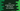# C++ program to find LCM of two numbers# Introduction:

In this C++ tutorial program, we will learn how to find the LCM of two user-given number. The program will take these numbers as input from the user and print out the result.

With this example, you will learn how to read user inputs, how to print values on the console, how to use an if-else condition and how to use a while loop in C++.

## LCM of two numbers :

LCM or lowest common multiplier of two numbers is the smallest number that is perfectly divisible by both numbers.

For example, LCM of 3 and 5 is 15.

## Algorithm :

To find out the LCM, we will use one loop that will run till the LCM is found. Suppose, we want to find out the LCM for 5 and 3. Following are the multipliers for each :

``````5 : 5, 10, 15, 20, 25....
3 : 3, 6, 9, 12, 15, 18, 21....
``````

So, if we are checking for an LCM, we can only check for the multiplier of the bigger number i.e. 5. If you check the multiplier or the smaller number or all numbers starting from 3, it will work. But the process will be faster if we check only the multiplier of the bigger number starting from 5 in this example.

The user will enter both numbers and our program will first verify which one is the bigger number. It will then keep checking for the LCM by using a loop.

## C++ program :

``````#include <iostream>
using namespace std;

int main()
{
//1
int firstNo, secondNo, lcm;
int count = 1;

//2
cout << "Enter the first number : " << endl; cin >> firstNo;

//3
cout << "Enter the second number : " << endl; cin >> secondNo;

//4
if (firstNo < secondNo)
{
//5
lcm = secondNo;

while (count < firstNo)
{
if (lcm % firstNo == 0 && lcm % secondNo == 0)
{
break;
}
lcm += secondNo;
count++;
}
}
else
{
//6
lcm = firstNo;
while (count < secondNo)
{
if (lcm % firstNo == 0 && lcm % secondNo == 0)
{
break;
}
lcm += firstNo;
count++;
}
}

//7
cout << "LCM is " << lcm << endl;

return 0;
}
``````

### Explanation :

The commented numbers in the above program denote the step numbers below :

1. firstNo, secondNo and lcm are three integer variables for holding the first number, second number and the LCM value.

2. Ask the user to enter the first number. Read and store it in firstNo variable.

3. Ask the user to enter the second number. Read and store it in secondNo variable.

4. Check if the firstNo is smaller than the secondNo or not. If yes, enter inside the if block or else enter in the else block.

5. If firstNo is smaller than secondNo, assign secondNo to lcm. Run one for loop until the value of count is less than firstNo. Check if the current value of lcm is divisible by both of these numbers or not. If yes, break from the loop or else increment the value of count and add secondNo to lcm.

The lcm variable is actually the multiplier or the bigger number. If the secondNo or the bigger number is 5, lcm will be 5, 10, 15… etc. The count will stop the operation once the value of lcm is equal to the product of both numbers. If no divisor is found, product of the two numbers will be the lowest common divisor always.

1. Similar to the step above, run one while loop and find the lcm if firstNo is greater than the secondNo.

2. Finally, print out the value of the LCM to the user.

### Sample Output :

``````Enter the first number :
3
Enter the second number :
5
LCM is 15

Enter the first number :
3
Enter the second number :
7
LCM is 21

Enter the first number :
100
Enter the second number :
10
LCM is 100

Enter the first number :
13
Enter the second number :
17
LCM is 221
``````

### Conclusion :

Go through the above program and try to run it on your system. If you have any queries, don’t hesitate to drop a comment below.